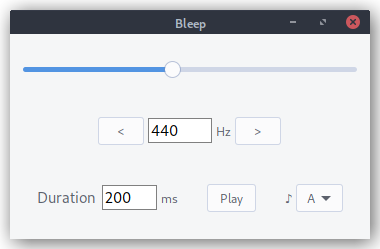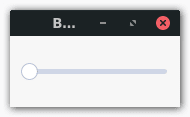# Part-Time Dragon Slayer

This tutorial was updated on January 10, 2021 to generate a tone using Racket instead of merely wrapping a Linux-only command-line program called beep that controls the PC speaker. This should now make it possible to follow this tutorial on any OS supported by Racket. This tutorial can also be found in the GitHub repo with the example code. Pull requests for improving the example and tutorial are welcome.

Racket is a Scheme-like dialect of Lisp that has a powerful cross-platform GUI library built in. Instead of building yet another calculator, let's build a GUI for generating a tone.You'll need Racket installed, of course. It's available in the repositories of most Linux distros, so just install it from your distro's repo. Then you're ready to begin.

``````#lang racket

(require racket/gui)
``````

One of the strengths of Racket is the number of built-in libraries. We'll be using the racket/gui library.

``````; Main window
(define frame (new frame% [label "Bleep"]))

; Display GUI
(send frame show #t)
``````

Racket's GUI library is object oriented. You create a window by instantiating the `frame%` class. Identifiers ending with a percent is Racket's naming convention for classes. You show the window by calling its `show` method. Now let's add some additional widgets between creating the window and showing it.

``````(define slider (new slider% [label #f]
[min-value 20]
[max-value 20000]
[parent frame]
[init-value 440]
[style '(horizontal plain)]
[vert-margin 25]
[horiz-margin 10]))
``````

The range of frequencies audible by humans is typically between 20 Hz and 20 KHz (we lose the ability to hear some of those higher frequencies as we age). The musical note A above middle C) is 440 Hz. Since A4 serves as a general tuning standard, it seems like a sensible default, but if you run the above in Racket, this is what you'll see:The scale of 20 to 20,000 is so large that 440 doesn't appear to move the slider at all. Ideally, 440 would fall about the middle of the slider. To achieve this, let's use a logarithmic scale.

I found a Stack Overflow answer on how to map a slider to a logarithmic scale. The code given in the answer is JavaScript, but it was easy enough to port to Racket.

``````; Scale used by slider
(define *min-position* 0)
(define *max-position* 2000)
; Range of frequencies
(define *min-frequency* 20)
(define *max-frequency* 20000)

; Logarithmic scale for frequency (so middle A  falls about in the middle)

(define min-freq (log *min-frequency*))
(define max-freq (log *max-frequency*))
(define frequency-scale (/ (- max-freq min-freq) (- *max-position* *min-position*)))
; Convert slider position to frequency
(define (position->frequency position)
(inexact->exact (round (exp (+ min-freq (* frequency-scale (- position *min-position*)))))))
; Convert frequency to slider position
(define (frequency->position freq)
(inexact->exact (round (/ (- (log freq) min-freq) (+ frequency-scale *min-position*)))))
``````

I added some global parameters to the top of the script. The variable name `*min-position*` is just a Lisp naming convention for global parameters. I came up with the range of 0-2,000 by trial and error. It seemed to strike the best balance between each step of the slider making a noticeable change to the frequency while still allowing the user to narrow in on a specific frequency with just the slider.

Then we create two functions: one that takes the position on the slider and returns the frequency (`position->frequency`) and another that takes a frequency and returns the position on the slider (`frequency-position`). Now let's modify our `slider%` to use `frequency->position` to convert the `init-value` to a slider position using our logarithmic scale.

``````(define slider (new slider% [label #f]
[min-value *min-position*]
[max-value *max-position*]
[parent frame]
[init-value (frequency->position 440)]
[style '(horizontal plain)]
[vert-margin 25]
[horiz-margin 10]))
``````

Underneath the slider is a text field showing the current frequency and buttons to increase/decrease the frequency by one octave.

``````(define frequency-pane (new horizontal-pane% [parent frame]
[border 10]
[alignment '(center center)]))
(define lower-button (new button% [parent frequency-pane]
[label "<"]))
(define frequency-field (new text-field% [label #f]
[parent frequency-pane]
[init-value "440"]
[min-width 64]
[stretchable-width #f]))
(define frequency-label (new message% [parent frequency-pane] [label "Hz"]))
(define higher-button (new button% [parent frequency-pane]
[label ">"]))
``````

The `horizontal-pane%` is an invisible widget that helps with layout. At this point, we are starting to have a nice looking interface, but it doesn't do anything. If you click the buttons or slide the slider, nothing happens. The widget classes accept a `callback` parameter that wires the widget up to a function. If we add a callback function to the slider, that function will be called each time the slider is moved.

``````; Link slider to text field display of frequency
(send frequency-field set-value
(~a (position->frequency (send widget get-value)))))
(define new-freq (string->number (send entry get-value)))
(send slider set-value
(frequency->position (if new-freq new-freq *min-frequency*))))
``````

A callback function takes two arguments: the first is the instance of the object that called it and the second is the event type. The `text-field%` expects a string, so we have to convert the number returned by `position->frequency` to a string with `~a`. Next all there is to do is wire these functions up to the widgets:

``````(define slider (new slider% [label #f]
...
...))
...
(define frequency-field (new text-field% [label #f]
...
...))
``````

Wire the buttons up to callback functions called `decrease-octave` and `increase-octave`. An octave is "the interval between one musical pitch and another with double its frequency."

``````; Set frequency slider and display
(define (set-frequency freq)
(send slider set-value (frequency->position freq))
(send frequency-field set-value (~a freq)))

; Buttons increase and decrease frequency by one octave
(set-frequency (* (string->number (send frequency-field get-value)) modifier)))
(define (decrease-octave button event) (adjust-octave 0.5))
(define (increase-octave button event) (adjust-octave 2))
``````

If you slide the slider, the text field updates accordingly. If you type a number in the text field, the slider updates accordingly. All good, right? What if a user (and you know they will) enters a number higher than 20,000 or a letter?

The widgets included with Racket are pretty basic, but we can extend the classes of the built-in widgets to create custom widgets. Let's extend the `text-field%` class to create a new `number-field%` class. This class will have two additional init variables that specify a `min-value` and `max-value` and only allow numbers that fall within that range.

``````; Extend the text-field% class to validate data when field loses focus. Field
; should contain only numbers within allowed range. Otherwise, set to min.
(define number-field%
(class text-field%
; Add init variables to define allowed range
(init min-value max-value)
(define min-allowed min-value)
(define max-allowed max-value)
(super-new)
(define/override (on-focus on?)
(unless on?
(define current-value (string->number (send this get-value)))
(unless (and current-value
(>= current-value min-allowed)
(<= current-value max-allowed))
(send this set-value (~a min-allowed))
; Also reset slider position to make sure it still matches display
(send slider set-value (string->number (send frequency-field get-value))))))))
``````

Then we can replace our `text-field%` with a `number-field%`.

``````(define frequency-field (new number-field% [label #f]
[parent frequency-pane]
[min-value *min-frequency*]
[max-value *max-frequency*]
[init-value "440"]
[min-width 64]
[stretchable-width #f]))
``````

Let's use this `number-field%` again to create a field to specify the duration of the beep in milliseconds:

``````(define control-pane (new horizontal-pane% [parent frame]
[border 25]
[spacing 25]))
(define duration-pane (new horizontal-pane% [parent control-pane]))
(define duration-field (new number-field% [label "Duration "]
[parent duration-pane]
[min-value 1]
[max-value 600000] ; 10 minutes
[init-value "200"]
[min-width 120]))
``````

Frequency is rather abstract. Let's also give the user the ability to select a musical note. We can store the corresponding frequencies for A4-G4 in a hash table.

``````; Notes -> frequency (middle A-G [A4-G4])
; http://pages.mtu.edu/~suits/notefreqs.html
(define notes (hash "A" 440.00
"B" 493.88
"C" 261.63
"D" 293.66
"E" 329.63
"F" 349.23
"G" 292.00))
``````

We'll give the user a drop-down menu. Whenever a note is selected from the drop-down menu, we'll look up the frequency in the hash table and set it using the `set-frequency` helper function we created for the octave buttons.

``````; Set frequency to specific note
(define (set-note choice event)
(set-frequency (hash-ref notes (send choice get-string-selection))))
(define note (new choice% [label "♪ "]
[choices '("A" "B" "C" "D" "E" "F" "G")]
[parent control-pane]
[callback set-note]))
``````

Finally, let's make some noise.

``````(require rsound)

; Generate a tone using RSound
; Explicitly set RSound sample rate in case differs by platform/version
(default-sample-rate 44100)
(define (generate-tone button event)
(play (make-tone (string->number (send frequency-field get-value))
0.5
; Duration in samples at sample rate of 44.1 kHz
(inexact->exact (* 44.1 (string->number (send duration-field get-value)))))))
``````

We'll use the Racket RSound package to generate the tone. This package isn't bundled with Racket, but you can install it with the `raco` utility that comes with Racket (```raco pkg install rsound```). Wire this up to a button between the duration and note selector, and you're ready to make some noise.

``````(define play-button (new button% [parent control-pane]
[label "Play"]
[callback generate-tone]))
``````

You can check out the entire example on GitHub. This started as a personal learning project to explore the state of GUI programming in Lisp and has become a series of tutorials on building GUIs with various dialects of Lisp.